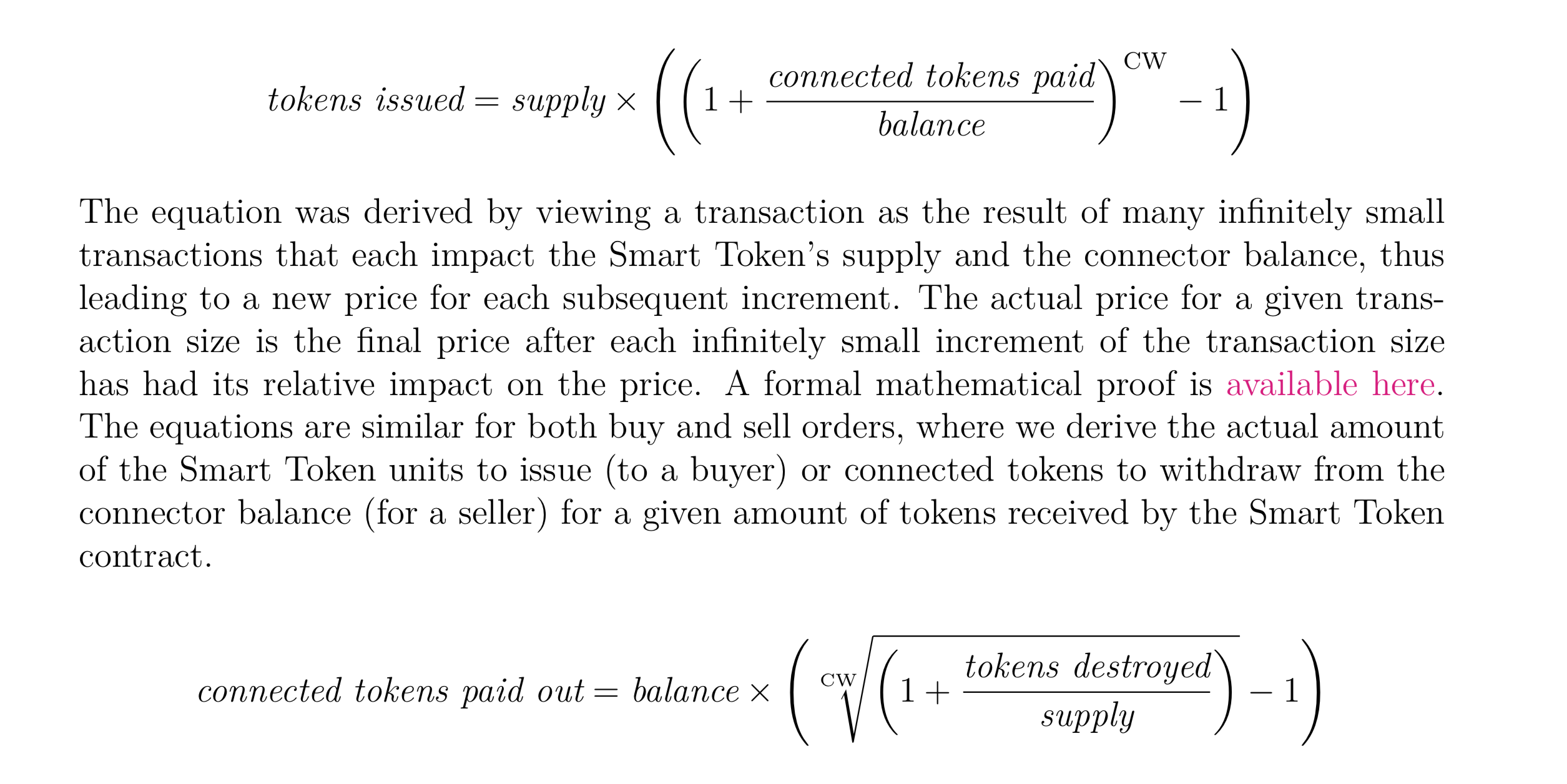## [EOSIO/eos] RAM price calculation does not match the formula in the Bancor Protocol paper (#4515)

Here is the formula in the [Bancor Protocol white paper](https://storage.googleapis.com/website-bancor/2018/04/01ba8253-bancor_protocol_whitepaper_en.pdf)In the formula, the balance and supply are the current value before the buy and sell action.

Here is the code from exchange_state.cpp.
«`
asset exchange_state::convert_to_exchange( connector& c, asset in ) {

real_type R(supply.amount);
real_type C(c.balance.amount+in.amount);
^^^^^^^^^^^^ This amount should not be added.
real_type F(c.weight/1000.0);
real_type T(in.amount);
real_type ONE(1.0);

real_type E = -R * (ONE — std::pow( ONE + T / C, F) );
//print( «E: «, E, «n»);
int64_t issued = int64_t(E);

supply.amount += issued;
c.balance.amount += in.amount;

return asset( issued, supply.symbol );
}

asset exchange_state::convert_from_exchange( connector& c, asset in ) {
eosio_assert( in.symbol== supply.symbol, «unexpected asset symbol input» );

real_type R(supply.amount — in.amount);
^^^^^^^^^^^^ This amount should not be subtracted.
real_type C(c.balance.amount);
real_type F(1000.0/c.weight);
real_type E(in.amount);
real_type ONE(1.0);

// potentially more accurate:
// The functions std::expm1 and std::log1p are useful for financial calculations, for example,
// when calculating small daily interest rates: (1+x)n
// -1 can be expressed as std::expm1(n * std::log1p(x)).
// real_type T = C * std::expm1( F * std::log1p(E/R) );

real_type T = C * (std::pow( ONE + E/R, F) — ONE);
//print( «T: «, T, «n»);
int64_t out = int64_t(T);

supply.amount -= in.amount;
c.balance.amount -= out;

return asset( out, c.balance.symbol );
}

«`
It seems the C in convert_to_exchange is not set according to the formula in the paper. The same for the R in convert_to_exchange.

Another question is about the setting of CW. Weight is set to 0.5. Why it is divided by 1000 again?
`F(c.weight/1000.0);`